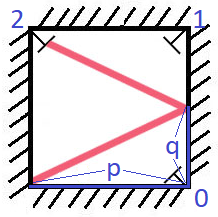## Algorithm

Problem Name: 858. Mirror Reflection

There is a special square room with mirrors on each of the four walls. Except for the southwest corner, there are receptors on each of the remaining corners, numbered `0`, `1`, and `2`.

The square room has walls of length `p` and a laser ray from the southwest corner first meets the east wall at a distance `q` from the `0th` receptor.

Given the two integers `p` and `q`, return the number of the receptor that the ray meets first.

The test cases are guaranteed so that the ray will meet a receptor eventually.

Example 1:```Input: p = 2, q = 1
Output: 2
Explanation: The ray meets receptor 2 the first time it gets reflected back to the left wall.
```

Example 2:

```Input: p = 3, q = 1
Output: 1
```

Constraints:

• `1 <= q <= p <= 1000`

## Code Examples

### #1 Code Example with Java Programming

```Code - Java Programming```

``````
class Solution {
public int mirrorReflection(int p, int q) {
int extention = q;
int reflection = p;
while (extention % 2 == 0 && reflection % 2 == 0) {
extention /= 2;
reflection /= 2;
}
if (extention % 2 == 0 && reflection % 2 != 0) {
return 0;
} else if (extention % 2 != 0 && reflection % 2 == 0) {
return 2;
} else {
return 1;
}
}
}
``````
Copy The Code &

Input

cmd
p = 2, q = 1

Output

cmd
2

### #2 Code Example with Javascript Programming

```Code - Javascript Programming```

``````
const mirrorReflection = function(p, q) {
while (p % 2 === 0 && q % 2 === 0) {
p /= 2;
q /= 2;
}

if (p % 2 === 0) {
return 2;
} else if (q % 2 === 0) {
return 0;
} else {
return 1;
}
};
``````
Copy The Code &

Input

cmd
p = 2, q = 1

Output

cmd
2

### #3 Code Example with Python Programming

```Code - Python Programming```

``````
class Solution:
def mirrorReflection(self, p, q):
side, up, h = 2, 1, 0
while True:
h += q * up
side = (side + 1) % 2
if side == 0:
side += 2
if h < 0:
h *= -1
up *= -1
elif h > p:
h = p - (h - p)
up *= -1
if h % p == 0:
return h and side or 0
``````
Copy The Code &

Input

cmd
p = 3, q = 1

Output

cmd
1